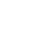# Next Homework Program: Ratio Exercise

The Badb just had a couple of weeks of reviewing ratios. This was a good limbering-up exercise, since the problem sets were all about converting between different units and manipulating fractions. After all, ratios are just a different way of writing fractions. The general form of the problems wound up being, “Given that the ratio of thingumbob : doohickii is γ, how many doohickii are there if there are N thingumbobs?” She’d have to set up the equation and solve for the doohickii quantity.

In the evenings, this was really not a problem (until she got really tired) but first thing in the morning it was a tough job, converting from decimals to fractions, simplifying fractions, and doing other straightforward (at 4 in the afternoon) operations. So let’s think about fractions for a minute. There are really two major operations one performs on fractions: multiplication and addition. Multiplication is easy (numerator times numerator, denominator times denominator, simplify, done) but addition is kind of a pain; you have to have a common denominator. Finding any common denominator is easy (heck, just multiply each denominator by the other) but we would prefer to work with the smallest whole numbers we can get away with, because smaller numbers are easier to handle and because humans, at least the smart ones, are lazy and hate doing extra work. So what we want, given two denominators A and B, is to find the least common multiple (LCM) of the two numbers.

Let’s say we want a program that lets you input two fractions as four integers (numerator A, denominator A, numerator B, denominator B) and it will output the original fractions, the required multipliers, and the multiplied fractions which have common denominators. That is, it’ll take something like this:

5/6

7/10

and give you

5/6 * 5/5 = 25/30

7/10 * 3/3 = 21/30

It should accept improper fractions (but not decimals and not mixed numbers). We’ve already got code that will spit out the prime factors of a number; I bet it will be useful here.

I play the bagpipes. I program computers. I support my family in their various endeavors, and I enjoy my wonderful life.

## 3 thoughts on “Next Homework Program: Ratio Exercise”

# Kurt-Werles-MacBook-Pro:/Users/kurt% ruby lcm_math.rb 5 6 7 10
# 5/6 * 5/5 = 25/30
#
# 7/10 * 3/3 = 21/30
#
# 25/30 + 21/30 = 46/30

numerator_a = ARGV.to_i
denominator_a = ARGV.to_i
numerator_b = ARGV.to_i
denominator_b = ARGV.to_i

lcm = denominator_a.lcm(denominator_b)
multiplier_a = lcm / denominator_a
multiplier_b = lcm / denominator_b

numerator_a_prime = numerator_a * multiplier_a
denominator_a_prime = denominator_a * multiplier_a

numerator_b_prime = numerator_b * multiplier_b
denominator_b_prime = denominator_b * multiplier_b # which had better be the same as denominator_a_prime

puts “#{numerator_a}/#{denominator_a} * #{multiplier_a}/#{multiplier_a} = #{numerator_a_prime}/#{denominator_a_prime}”

puts “”

puts “#{numerator_b}/#{denominator_b} * #{multiplier_b}/#{multiplier_b} = #{numerator_b_prime}/#{denominator_b_prime}”

puts “”

puts “#{numerator_a_prime}/#{denominator_a_prime} + #{numerator_b_prime}/#{denominator_b_prime} = #{numerator_a_prime + numerator_b_prime}/#{denominator_b_prime}”

# I love ruby. lcm. Who knew?

1.pirateguillermo – Santa Cruz, CA – I play the bagpipes. I program computers. I support my family in their various endeavors, and I enjoy my wonderful life. pirateguillermo says:

I think that’s very cool. It raises a couple of questions, though:

1) In all the years you and I have been doing professional software development, have either of us ever needed to find the lowest common denominator or the greatest common factor (heck, even the prime factors) of a pair of numbers? I haven’t. So why does that admittedly cool function exist?

2) You’ve reminded me of the caution of evolutionary programming: your algorithm will optimize for the fitness criteria, so be careful how you express those criteria. What’s the point of the homework? Does the teacher have a burning desire to know the sum of 5/6 and 7/15? Clearly, it’s not really a quest for arithmetic answers. What is it a quest for? Probably, it’s an exercise in repetition because human brains don’t really learn things until they’ve been repeated several times. And since it’s not the same problem over and over, it’s not an exercise in memorizing the LCM of 6 and 15. It’s probably an exercise in memorizing the techniques of manipulating fractions. Why would we need to do that, since there’s this swell LCM function? I dunno, maybe to get the kid ready to work on algebraic expressions that involve fractions without freaking out.

3) Why didn’t you do it in BASIC?